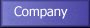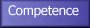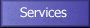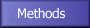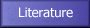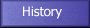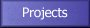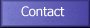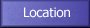# Definition of an MINLP Problem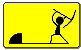For xT=(x1,...,x_{n_c}) and yT=(y1,...,ynd), objective function f(x,y) and constraints g(x,y) and h(x,y) an optimization problem (*)
min f(x,y)
subject to
g(x,y)=0 , h(x,y)>0
is called a mixed integer nonlinear programming problem (MINLP), if the domain U is discrete, e.g., U=\bbbn0={0,1,2,3,...} and the functions f(x,y), g(x,y) and h(x,y) are nonlinear.

The continuous variables in (*) could for instance describe the
states (temperature, pressure, etc.), flow rates or design parameters of
plant or chemical reactors. The discrete variables, often binary variables,
may be used to describe the topology of a process network or to represent
the existence or non-existence of plants. Mostly the binary variables appear
only linearly. Let us consider the following pure integer nonlinear problem
[a feasible solution is (y1,y2)=(3,66), for instance]:

\min_{y1,y2}\ \ \left\{ \ 3y1+2y22
s.t.
y14-y2-15=0 \\
y1+y2-3>= 0          y1,y2\in \bbbn_0.
The optimal solution is y*=(y1,y2)*=(2,1) and f(y)=8.

#### Some General Comments on MINLP

MINLP problems such as (*) are the most difficult optimisation problems of all. They belong to the class NP-complete problems, i.e., at present no algorithm is known which could solve (*) in polynomial time (polynomial in the problem size).

Mixed integer linear programming (MILP) problems are combinatorial optimization problems for which Branch&Bound (B&B) with LP
relaxation proves to be sufficiently efficient in many cases. Nonlinear programming (NLP) problems cannot be solved in a finite number of steps but only iteratively. Nevertheless, solving NLP problems is usually easier than
solving MILP problems.

Unfortunately, MINLP problems combine all the difficulties of both its subclasses: MILP and NLP. In addition they have properties absent in NLP or MILP. While for convex NLP problems a local minimum is identical to the
global minimum, this result does not hold for MINLP problems. At present
there exists no algorithm which could solve (*) in its general form exactly. Therefore, only special instances of (*) are investigated. A significant assumption is convexity.

Solution algorithms in discrete optimization belong to two classes: deterministic (or exact) and heuristic methods. All deterministic methods use implicit enumeration and tree search rules and they can decide whether a given solution is optimal or not. Heuristic methods on its own lack this feature. Unfortunately, for non-convex problems no efficient deterministic methods are known.

A simple deterministic method is to list all combinations U of discrete variables yi. Each binary vector yi generates an NLP in the continuous variable vector x. If we solve this NLP problem, it is either infeasible or yields a solution, i.e., a pair (xi,zi=f(xi,yi)). After having solved all NLP problems, we choose the pair with smallest zi (let us refer to it using the index i*). Thus, the solution is given by the triple (x*=xi*,y*=yi*,zi*=f(xi*,yi*)). This method, of course, works only if U has a finite number of elements and if the NLP subproblems allow us to determine their global minima. For most problems this method is of no practical use because of the prohibitively high numerical effort.

#### Deterministic Methods for Solving MINLP Problems

Deterministic methods for solving (convex) MINLP problems fall into three
classes:

1. Branch \& Bound by Gupta and Ravindran (1985; GR85 hereafter),

2. Generalised Benders Decomposition (GBD) by Geoffrion (1972; Ge72
hereafter) and

3. Outer Approximation (OA) by Duran and Grossmann (1986; DG86 hereafter).

The B&B algorithm for MINLP problems by GR85 is based on the same
ideas as B&B for solving MILP problems. The first step is to solve the problem generated by relaxing the integrality condition on the variables. If the solution of that problem fulfils all integrality conditions the whole problems is solved. Otherwise, in a minimization problem the relaxed problem provides a lower bound (of course only if the global minimum can be determined) and the search tree is built up. A feasible integer solution provides an upper bound. A major drawback of B&B applied to MINLP problems is that nodes deeper in the tree cannot benefit so greatly from information available at previous nodes as is the case in MILP B&B using the dual simplex algorithm.

Generalised Benders Decomposition divides the variables into two sets:
complicating and non-complicating variables. In MINLP models the class of
complicating variables is made up by the discrete (usually binary) variables. Then the algorithm generates a sequence of NLP sub-problems (produced by fixing the binary variables yk) and solves the so-called MILP Master problems in the space of the complicating variables. The NLP sub-problems yield upper bounds for the original problem while the MILP Master problems yield additional combination of binary variables yk for subsequent NLP sub-problems. Under convexity assumptions the Master problems generate a sequence of lower bounds increasing monotonically. The algorithm terminates if lower and upper bounds equal or cross each other.

Outer Approximation also consists of a sequence of NLP sub-problems (produced by fixing the binary variables yk generated by MILP Master problems. The significant difference is how the Master problems are defined. Algorithms based on OA describe the feasible region as the intersection of an infinite collection of sets with a simpler structure, e.g., polyhedra. In OA the Master problems are generated by "outer approximations'' (linearisations, or Taylor series expansions) of the nonlinear constraints in those points which are the optimal solutions of the NLP subproblems; that is, a finite collection of sets. The key idea is to solve the MINLP with a much smaller set of points, i.e., tangential planes. In convex MINLP problems, a superset of the feasible
region is established. Thus, the OA Master problems (MILP problem in both
discrete and continuous variables) produce a sequence of increasing lower
bounds. The termination criterion is the same as above.

While the GBD Master problems have fewer variables and constraints, the OA algorithm provides tighter bounds and needs fewer iterations for
convergence. Both algorithms have heuristic extensions for non-convex MINLP. In many instances they are even capable of proving optimality.

If you have any questions to MaBOS GmbH please send us an e-mail to info@mabos.com.

If there are any network problems please mail the problem to the local Webmaster of MaBOS.